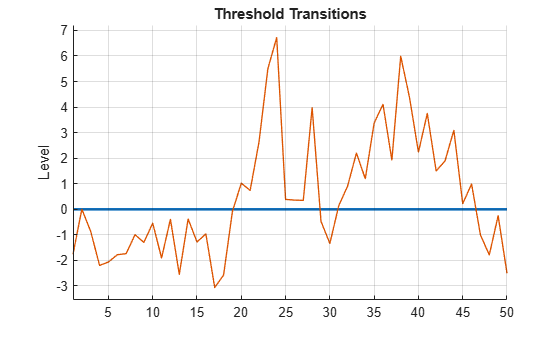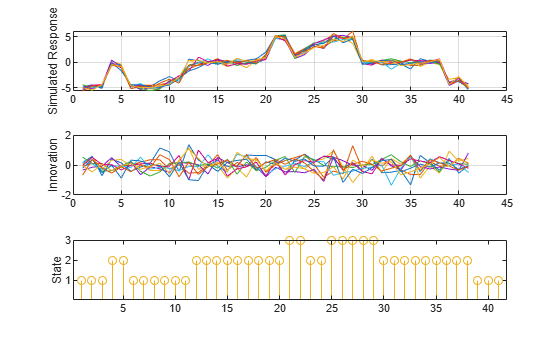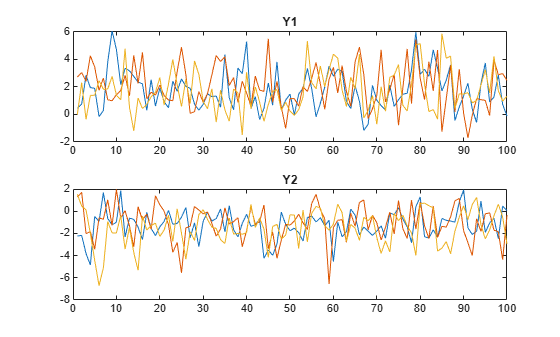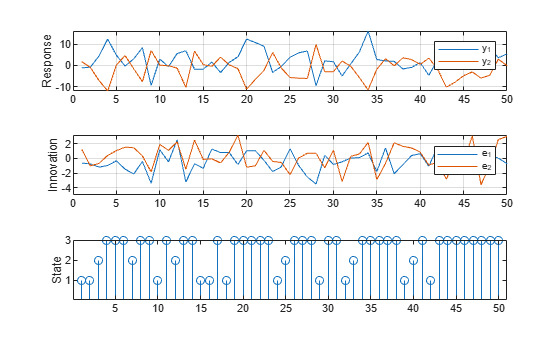# simulate

Simulate sample paths of threshold-switching dynamic regression model

## Syntax

``Y = simulate(Mdl,numObs)``
``Y = simulate(Mdl,numObs,Name,Value)``
``[Y,E,StatePaths] = simulate(___)``

## Description

example

````Y = simulate(Mdl,numObs)` returns a random `numObs`-period path of response series `Y` from simulating the fully specified threshold-switching dynamic regression model `Mdl`.```

example

````Y = simulate(Mdl,numObs,Name,Value)` uses additional options specified by one or more name-value arguments. For example, `simulate(Mdl,10,NumPaths=1000,Y0=Y0)` simulates `1000` sample paths of length `10`, and initializes the dynamic component of each submodel by using the presample response data `Y0`.```

example

````[Y,E,StatePaths] = simulate(___)` also returns the simulated innovation paths `E` and the simulated state paths `StatePaths`, using any of the input argument combinations in the previous syntaxes. ```

## Examples

collapse all

Suppose a data-generating process (DGP) is a two-state, self-exciting threshold autoregressive (SETAR) model for a 1-D response variable. Specify all parameter values (this example uses arbitrary values).

Create Fully Specified Model for DGP

Create a discrete threshold transition at level 0. Label the regimes to reflect the state of the economy:

When the threshold variable (currently unknown) is in $\left(-\infty ,0\right)$, the economy is in a recession.

When the threshold variable is in $\left[0,\infty \right)$, the economy is expanding.

```t = 0; tt = threshold(t,StateNames=["Recession" "Expansion"])```
```tt = threshold with properties: Type: 'discrete' Levels: 0 Rates: [] StateNames: ["Recession" "Expansion"] NumStates: 2 ```

`tt` is a fully specified `threshold` object that describes the switching mechanism of the threshold-switching model.

Assume the following univariate models describe the response process of the system:

• Recession: ${\mathit{y}}_{\mathit{t}}=-1+0.1{\mathit{y}}_{\mathit{t}-1}+{\epsilon }_{1,\mathit{t}}$, where ${\epsilon }_{1,\mathit{t}}\sim Ν\left(0,1\right)$.

• Expansion: ${\mathit{y}}_{\mathit{t}}=1++0.3{\mathit{y}}_{\mathit{t}-1}+0.2{\mathit{y}}_{\mathit{t}-2}+{\epsilon }_{2,\mathit{t}}$, where ${\epsilon }_{2,\mathit{t}}\sim Ν\left(0,{2}^{2}\right)$.

For each regime, use `arima` to create an AR model that describes the response process within the regime.

```c1 = -1; c2 = 1; ar1 = 0.1; ar2 = [0.3 0.2]; v1 = 1; v2 = 4; mdl1 = arima(Constant=c1,AR=ar1,Variance=v1,... Description="Recession State Model")```
```mdl1 = arima with properties: Description: "Recession State Model" Distribution: Name = "Gaussian" P: 1 D: 0 Q: 0 Constant: -1 AR: {0.1} at lag  SAR: {} MA: {} SMA: {} Seasonality: 0 Beta: [1×0] Variance: 1 ARIMA(1,0,0) Model (Gaussian Distribution) ```
```mdl2 = arima(Constant=c2,AR=ar2,Variance=v2,... Description="Expansion State Model")```
```mdl2 = arima with properties: Description: "Expansion State Model" Distribution: Name = "Gaussian" P: 2 D: 0 Q: 0 Constant: 1 AR: {0.3 0.2} at lags [1 2] SAR: {} MA: {} SMA: {} Seasonality: 0 Beta: [1×0] Variance: 4 ARIMA(2,0,0) Model (Gaussian Distribution) ```

`mdl1` and `mdl2` are fully specified `arima` objects.

Store the submodels in a vector with order corresponding to the regimes in `tt.StateNames`.

`mdl = [mdl1; mdl2];`

Use `tsVAR` to create a TAR model from the switching mechanism `tt` and the state-specific submodels `mdl`.

`Mdl = tsVAR(tt,mdl)`
```Mdl = tsVAR with properties: Switch: [1x1 threshold] Submodels: [2x1 varm] NumStates: 2 NumSeries: 1 StateNames: ["Recession" "Expansion"] SeriesNames: "1" Covariance: [] ```
`Mdl.Submodels(2)`
```ans = varm with properties: Description: "AR-Stationary 1-Dimensional VAR(2) Model" SeriesNames: "Y1" NumSeries: 1 P: 2 Constant: 1 AR: {0.3 0.2} at lags [1 2] Trend: 0 Beta: [1×0 matrix] Covariance: 4 ```

`Mdl` is a fully specified `tsVAR` object representing a univariate two-state TAR model. `tsVAR` stores specified `arima` submodels as `varm` objects.

Simulate Response Data from DGP

Generate one random response path of length 50 from the model. `simulate` assumes that the threshold variable is ${\mathit{y}}_{\mathit{t}-1}$, which implies that the model is self-exciting.

```rng(1); % For reproducibility y = simulate(Mdl,50);```

`y` is a 50-by-1 vector of one response path.

Plot the response path with the threshold by using `ttplot`.

```figure ttplot(Mdl.Switch,Data=y)```Consider the following logistic TAR (LSTAR) model for the annual, CPI-based, Canadian inflation rate series ${\mathit{y}}_{\mathit{t}}$.

• State 1: ${\mathit{y}}_{\mathit{t}}=-5+{\epsilon }_{1,\mathit{t}}$, where ${\epsilon }_{1,\mathit{t}}\sim Ν\left(0,0.{1}^{2}\right).$

• State 2: ${\mathit{y}}_{\mathit{t}}={\epsilon }_{2,\mathit{t}}$, where ${\epsilon }_{2,\mathit{t}}\sim Ν\left(0,0.{2}^{2}\right).$

• State 3: ${\mathit{y}}_{\mathit{t}}=5+{\epsilon }_{3,\mathit{t}}$, where ${\epsilon }_{3,\mathit{t}}\sim Ν\left(0,0.{3}^{2}\right).$

• The system is in state 1 when ${\mathit{y}}_{\mathit{t}}<2$, the system is in state 2 when $2\le {\mathit{y}}_{\mathit{t}}<8$, and the system is in state 3 otherwise.

• The transition function rate between states 1 and 2 is 3.5, and the transition function rate between states 2 and 3 is 1.5.

Create an LSTAR model representing ${\mathit{y}}_{\mathit{t}}$.

```t = [2 8]; tt = threshold([2 8],Type="logistic",Rates=[3.5 1.5]); mdl1 = arima(Constant=-5,Variance=0.1); mdl2 = arima(Constant=0,Variance=0.2); mdl3 = arima(Constant=5,Variance=0.3); Mdl = tsVAR(tt,[mdl1; mdl2; mdl3]);```

`load Data_Canada`

Extract the CPI-based inflation rate series.

```INF_C = DataTable.INF_C; numObs = length(INF_C);```

Simulate ten paths from the model. Specify the threshold variable type and its data.

`Y = simulate(Mdl,numObs,NumPaths=10,Type="exogenous",Z=INF_C);`

Y is a `numObs`-by-10 matrix of simulated paths. Each column represents an independently simulated path.

In a tiled layout, plot the threshold transitions with the data by using `ttplot`,and plot the simulated paths to one tile.

```tiledlayout(2,1) nexttile ttplot(tt,Data=INF_C) colorbar('off') xticklabels(dates(xticks)) nexttile plot(dates,Y) grid on axis tight title("Simulations")````Y` switches between submodels according to the value of the threshold variable `INF_C`. Mixing is evident for observations near thresholds, such as at the inflation rates of 1964 and 1978.

Consider the model for the annual, CPI-based, Canadian inflation rate series in Simulate Multiple Paths.

Create the LSTAR model for the series.

```t = [2 8]; tt = threshold([2 8],Type="logistic",Rates=[3.5 1.5]); mdl1 = arima(Constant=-5,Variance=0.1); mdl2 = arima(Constant=0,Variance=0.2); mdl3 = arima(Constant=5,Variance=0.3); Mdl = tsVAR(tt,[mdl1; mdl2; mdl3]);```

Load the Canadian inflation and interest rate data set and extract the inflation rate series.

```load Data_Canada INF_C = DataTable.INF_C; numObs = length(INF_C);```

Simulate a length `numObs` path from the model. Specify the threshold variable type and its data. Return the innovations and states.

```[y,e,s] = simulate(Mdl,numObs,NumPaths=10,Type="exogenous",Z=INF_C); tiledlayout(3,1) nexttile plot(y); ylabel("Simulated Response") grid on nexttile plot(e) ylabel('Innovation') grid on nexttile stem(s) ylabel('State') yticks([1 2 3]) yticklabels(Mdl.StateNames)```This example shows how to initialize simulated paths from presample responses and initial states. The example uses arbitrary parameter values.

Fully Specify LSETAR Model

Consider the following 2-D LSETAR model.

• State 1, `"Low"`: ${y}_{t}=\left[\begin{array}{c}1\\ -1\end{array}\right]+{\epsilon }_{1,t},$ where ${\epsilon }_{1,t}\sim N\left(\left[\begin{array}{c}0\\ 0\end{array}\right],\left[\begin{array}{cc}1& -0.1\\ -0.1& 1\end{array}\right]\right).$

• State 2 , `"Med"`: ${y}_{t}=\left[\begin{array}{c}2\\ -2\end{array}\right]+\left[\begin{array}{cc}0.5& 0.1\\ 0.5& 0.5\end{array}\right]{y}_{t-1}+{\epsilon }_{2,t},$ where ${\epsilon }_{2,t}\sim N\left(\left[\begin{array}{c}0\\ 0\end{array}\right],\left[\begin{array}{cc}2& -0.2\\ -0.2& 2\end{array}\right]\right).$

• State 3, `"High"`: ${y}_{t}=\left[\begin{array}{c}3\\ -3\end{array}\right]+\left[\begin{array}{cc}0.25& 0\\ 0& 0\end{array}\right]{y}_{t-1}+\left[\begin{array}{cc}0& 0\\ 0.25& 0\end{array}\right]{y}_{t-2}+{\epsilon }_{3,t},$ where ${\epsilon }_{3,t}\sim N\left(\left[\begin{array}{c}0\\ 0\end{array}\right],\left[\begin{array}{cc}3& -0.3\\ -0.3& 3\end{array}\right]\right).$

• The system is in state 1 when ${\mathit{y}}_{2,\mathit{t}-4}<-1$, the system is in state 2 when $-1\le {\mathit{y}}_{2,\mathit{t}-4}<1$, and the system is in state 3 otherwise.

• The transition function is logistic. The transition rate from state 1 to 2 is 3.5, and the transition rate from state 1 to 3 is 1.5.

Create logistic threshold transitions at mid-levels -1 and 1 with rates `3.5` and `1.5`, respectively. Label the states.

```t = [-1 1]; r = [3.5 1.5]; stateNames = ["Low" "Med" "High"]; tt = threshold(t,Type="logistic",Rates=[3.5 1.5],StateNames=stateNames);```

Create the VAR submodels by using `varm`. Store the submodels in a vector with order corresponding to the regimes in `tt.StateNames`.

```% Constants (numSeries x 1 vectors) C1 = [1; -1]; C2 = [2; -2]; C3 = [3; -3]; % Autoregression coefficients (numSeries x numSeries matrices) AR1 = {}; % 0 lags AR2 = {[0.5 0.1; 0.5 0.5]}; % 1 lag AR3 = {[0.25 0; 0 0] [0 0; 0.25 0]}; % 2 lags % Innovations covariances (numSeries x numSeries matrices) Sigma1 = [1 -0.1; -0.1 1]; Sigma2 = [2 -0.2; -0.2 2]; Sigma3 = [3 -0.3; -0.3 3]; % VAR Submodels mdl1 = varm('Constant',C1,'AR',AR1,'Covariance',Sigma1); mdl2 = varm('Constant',C2,'AR',AR2,'Covariance',Sigma2); mdl3 = varm('Constant',C3,'AR',AR3,'Covariance',Sigma3); mdl = [mdl1; mdl2; mdl3];```

Create an LSETAR model from the switching mechanism `tt` and the state-specific submodels `mdl`. Label the series `Y1` and `Y2`.

`Mdl = tsVAR(tt,mdl,SeriesNames=["Y1" "Y2"])`
```Mdl = tsVAR with properties: Switch: [1x1 threshold] Submodels: [3x1 varm] NumStates: 3 NumSeries: 2 StateNames: ["Low" "Med" "High"] SeriesNames: ["Y1" "Y2"] Covariance: [] ```

`Mdl` is a fully specified `tsVAR` object representing a multivariate three-state LSETAR model. `tsVAR` object functions enable you to specify threshold variable characteristics and data.

Initialize Simulation from Presample Responses

Consider simulating 5 paths initialized from presample responses. Specify a `numPreObs`-by-`numSeries`-by-`numPaths` array of presample responses, where:

• `numPreObs` is the number of presample responses per series and path. You must specify enough presample observations to initialize all AR components in the VAR models and the endogenous threshold variable. The largest AR component order is 2 and the threshold variable delay is 4, therefore `simulate` requires `numPreObs=4` presample observations per series and path.

• `numSeries=2`, the number of response series in the system.

• `numPaths=5`, the number of independent paths to simulate.

```delay = 4; numPaths = 5; Y0 = zeros(delay,Mdl.NumSeries,numPaths); for j = 2:numPaths Y0(:,:,j) = 10*j*ones(delay,Mdl.NumSeries); end```

Simulate 10 paths of length 100 from the LSETAR model from the presample. Specify the endogenous threshold variable and its delay, ${\mathit{y}}_{2,\mathit{t}-4}$.

```numObs = 100; rng(1); Y = simulate(Mdl,numObs,NumPaths=numPaths,Y0=Y0,Index=2,Delay=4);```

Y is a 100-by-2-by-5 array of simulate response paths. For example, `Y(50,2,3)` is the simulated response of path `3`, of series `Y2`, at time point `50`.

Plot the simulated paths for each variable on separate plots.

```tiledlayout(2,1) nexttile plot(squeeze(Y(:,1,:))) title("Y1") nexttile plot(squeeze(Y(:,2,:))) title("Y2")```The system quickly settles regardless of the presample.

Initialize Simulation from States

Simulate three paths of length 100, where each of the three states initialize a path. Specify state indices for initialization, and specify the endogenous threshold variable and its delay.

```S0 = 1:Mdl.NumStates; numPaths = numel(S0); Y = simulate(Mdl,numObs,NumPaths=numPaths,S0=S0,Index=2,Delay=4); tiledlayout(2,1) nexttile plot(squeeze(Y(:,1,:))) title("Y1") nexttile plot(squeeze(Y(:,2,:))) title("Y2")```Consider including regression components for exogenous variables in each submodel of the threshold-switching dynamic regression model in Initialize Multivariate Model Simulation from Multiple Starting Conditions.

Fully Specify LSETAR Model

Create logistic threshold transitions at mid-levels `-1` and `1` with rates `3.5` and `1.5`, respectively. Label the states.

```t = [-1 1]; r = [3.5 1.5]; stateNames = ["Low" "Med" "High"]; tt = threshold(t,Type="logistic",Rates=[3.5 1.5],StateNames=stateNames)```
```tt = threshold with properties: Type: 'logistic' Levels: [-1 1] Rates: [3.5000 1.5000] StateNames: ["Low" "Med" "High"] NumStates: 3 ```

Assume the following VARX models describe the response processes of the system:

• State 1: ${y}_{t}=\left[\begin{array}{c}1\\ -1\end{array}\right]+\left[\begin{array}{c}1\\ -1\end{array}\right]{x}_{1,t}+{\epsilon }_{1,t},$ where ${\epsilon }_{1,t}\sim N\left(\left[\begin{array}{c}0\\ 0\end{array}\right],\left[\begin{array}{cc}1& -0.1\\ -0.1& 1\end{array}\right]\right).$

• State 2: ${y}_{t}=\left[\begin{array}{c}2\\ -2\end{array}\right]+\left[\begin{array}{cc}2& 2\\ -2& -2\end{array}\right]{x}_{2,t}+\left[\begin{array}{cc}0.5& 0.1\\ 0.5& 0.5\end{array}\right]{y}_{t-1}+{\epsilon }_{2,t},$ where ${\epsilon }_{2,t}\sim N\left(\left[\begin{array}{c}0\\ 0\end{array}\right],\left[\begin{array}{cc}2& -0.2\\ -0.2& 2\end{array}\right]\right).$

• State 3: ${y}_{t}=\left[\begin{array}{c}3\\ -3\end{array}\right]+\left[\begin{array}{ccc}3& 3& 3\\ -3& -3& -3\end{array}\right]{x}_{3,t}+\left[\begin{array}{cc}0.25& 0\\ 0& 0\end{array}\right]{y}_{t-1}+\left[\begin{array}{cc}0& 0\\ 0.25& 0\end{array}\right]{y}_{t-2}+{\epsilon }_{3,t},$ where ${\epsilon }_{3,t}\sim N\left(\left[\begin{array}{c}0\\ 0\end{array}\right],\left[\begin{array}{cc}3& -0.3\\ -0.3& 3\end{array}\right]\right).$

${\mathit{x}}_{1,\mathit{t}}$ represents a single exogenous variable, ${\mathit{x}}_{2,\mathit{t}}$ represents two exogenous variables, and ${\mathit{x}}_{3,\mathit{t}}$ represents three exogenous variables. Store the submodels in a vector.

```% Constants (numSeries x 1 vectors) C1 = [1; -1]; C2 = [2; -2]; C3 = [3; -3]; % Regression coefficients (numSeries x numRegressors matrices) Beta1 = [1; -1]; % 1 regressor Beta2 = [2 2; -2 -2]; % 2 regressors Beta3 = [3 3 3; -3 -3 -3]; % 3 regressors % Autoregression coefficients (numSeries x numSeries matrices) AR1 = {}; AR2 = {[0.5 0.1; 0.5 0.5]}; AR3 = {[0.25 0; 0 0] [0 0; 0.25 0]}; % Innovations covariances (numSeries x numSeries matrices) Sigma1 = [1 -0.1; -0.1 1]; Sigma2 = [2 -0.2; -0.2 2]; Sigma3 = [3 -0.3; -0.3 3]; %VARX submodels mdl1 = varm(Constant=C1,AR=AR1,Beta=Beta1,Covariance=Sigma1); mdl2 = varm(Constant=C2,AR=AR2,Beta=Beta2,Covariance=Sigma2); mdl3 = varm(Constant=C3,AR=AR3,Beta=Beta3,Covariance=Sigma3); mdl = [mdl1; mdl2; mdl3];```

Create an LSETAR model from the switching mechanism `tt` and the state-specific submodels `mdl`. Label the series `Y1` and `Y2`.

`Mdl = tsVAR(tt,mdl,SeriesNames=["Y1" "Y2"])`
```Mdl = tsVAR with properties: Switch: [1x1 threshold] Submodels: [3x1 varm] NumStates: 3 NumSeries: 2 StateNames: ["Low" "Med" "High"] SeriesNames: ["Y1" "Y2"] Covariance: [] ```

Simulate Data Ignoring Regression Component

If you do not supply exogenous data, `simulate` ignores the regression components in the submodels. Simulate a single path of responses, innovations, and states into a simulation horizon of length 50. Then plot each path separately.

```rng(1); % For reproducibility numObs = 50; [Y,E,SP] = simulate(Mdl,numObs); figure tiledlayout(3,1) nexttile plot(Y) ylabel("Response") grid on legend(["y_1" "y_2"]) nexttile plot(E) ylabel("Innovation") grid on legend(["e_1" "e_2"]) nexttile stem(SP) ylabel("State") yticks([1 2 3])```Simulate Data Including Regression Component

`simulate` requires exogenous data in order to generate random paths from the model. Simulate exogenous data for the three regressors by generating 50 random observations from the 3-D standard Gaussian distribution.

`X = randn(50,3);`

Generate one random response, innovation, and state path of length 50. Specify the simulated exogenous data for the submodel regression components. Plot the results.

```rng(1); % Reset seed for comparison [Y,E,SP] = simulate(Mdl,numObs,X=X); figure tiledlayout(3,1) nexttile plot(Y) ylabel("Response") grid on legend(["y_1" "y_2"]) nexttile plot(E) ylabel("Innovation") grid on legend(["e_1" "e_2"]) nexttile stem(SP) ylabel("State") yticks([1 2 3])```This example shows how to use Monte Carlo estimation to obtain an interval estimate of the threshold mid-level.

Consider a SETAR model for the real US GDP growth rate ${\mathit{y}}_{\mathit{t}}$ with AR(4) submodels. Suppose the threshold variable is ${\mathit{y}}_{\mathit{t}}$ (self exciting with 0 delay).

Create a discrete threshold transition at unknown mid-level ${\mathit{t}}_{1}$. Label the states `"Recession"` and `"Expansion"`.

`tt = threshold(NaN,StateNames=["Recession" "Expansion"]);`

For each state, create a partially specified AR(4) model with one coefficient at lag 4. Store the state submodels in a vector.

```submdl = arima(ARLags=4); mdl = [submdl; submdl];```

Each submodel has an unknown, estimable lag 4 coefficient, model constant, and innovations variance.

Create a partially specified TAR model from the threshold transition and submodel vector.

`Mdl = tsVAR(tt,mdl);`

Create a fully specified threshold transition that has the same structure as `tt`, but set the mid-level to `0`.

`tt0 = threshold(0);`

Load the US macroeconomic data set. Compute the real GDP growth rate as a percent.

```load Data_USEconModel rGDP = DataTable.GDP./DataTable.GDPDEF; pRGDP = 100*price2ret(rGDP); T = numel(pRGDP);```

Fit the TAR to the real GDP rate series.

`EstMdl = estimate(Mdl,tt0,pRGDP,Z=pRGDP,Type="exogenous");`

Simulate 100 response paths from the estimated model.

```rng(100) % For reproducibility numPaths= 100; Y = simulate(EstMdl,T,NumPaths=numPaths,Z=pRGDP,Type="exogenous");```

Fit the TAR model to each simulated response path. Specify the estimated threshold transition `EstMdl.Switch` to initialize the estimation procedure. For each path, store the estimated threshold transition mid-level.

```tMC = nan(T,1); for j = 1:numPaths EstMdlSim = estimate(Mdl,EstMdl.Switch,Y(:,j),Z=Y(:,j),Type="exogenous"); tMC(j) = EstMdlSim.Switch.Levels; end```

`tMC` is a 100-by-1 vector representing a Monte Carlo sample of threshold transitions.

Obtain a 95% confidence interval on the true threshold transition by computing the 0.25 and .975 quantiles of the Monte Carlo sample.

`tCI = quantile(tMC,[0.025 0.975])`
```tCI = 1×2 0.5158 0.9810 ```

A 95% confidence interval on the true threshold transition is (0.52%, 0.98%).

## Input Arguments

collapse all

Fully specified threshold-switching dynamic regression model, specified as an `tsVAR` model object returned by `tsVAR` or `estimate`. Properties of a fully specified model object do not contain `NaN` values.

Number of observations to generate for each sample path, specified as a positive integer.

Data Types: `double`

### Name-Value Arguments

Specify optional pairs of arguments as `Name1=Value1,...,NameN=ValueN`, where `Name` is the argument name and `Value` is the corresponding value. Name-value arguments must appear after other arguments, but the order of the pairs does not matter.

Before R2021a, use commas to separate each name and value, and enclose `Name` in quotes.

Example: `NumPaths=1000,Y0=Y0` simulates `1000` sample paths and initializes the dynamic component of each submodel by using the presample response data `Y0`.

Number of sample paths to generate, specified as a positive integer.

Example: `NumPaths=1000`

Data Types: `double`

Type of threshold variable data, specified as a value in this table.

ValueDescription
`"endogenous"`

The model is self-exciting with threshold variable data ${z}_{t}={y}_{j,\left(t-d\right)},$ generated by response j, where

`"exogenous"`The threshold variable is exogenous to the system. The name-value argument `'Z'` specifies the threshold variable data and is required.

Example: `Type="exogenous",Z=z` specifies the data `z` for the exogenous threshold variable.

Example: `Type="endogenous",Index=2,Delay=4` specifies the endogenous threshold variable as y2,t−4, whose data is `Y(:,2)`.

Data Types: `char` | `string` | `cell`

Presample response data, specified as a numeric matrix or array.

To use the same presample data for each of the `numPaths` path, specify a `numPreSampleObs`-by-`numSeries` matrix, where `numPaths` is the value of `NumPaths`, `numPreSampleObs` is the number of presample observations, and `numSeries` is the number of response variables.

To use different presample data for each path:

• For univariate ARX submodels, specify a `numPreSampleObs`-by-`numPaths` matrix.

• For multivariate VARX submodels, specify a `numPreSampleObs`-by-`numSeries`-by-`numPaths` array.

The number of presample observations `numPreSampleObs` must be sufficient to initialize the AR terms of all submodels. For models of type `"endogenous"`, the number of presample observations must also be sufficient to initialize the delayed response. If `numPreSampleObs` exceeds the number necessary to initial the model, `simulate` uses only the latest observations. The last row contains the latest observations.

`simulate` updates `Y0` using the latest simulated observations each time it switches states.

By default, `simulate` determines `Y0` by the submodel of the initial state:

• If the initial submodel is a stationary AR process without regression components, `simulate` sets presample observations to the unconditional mean.

• Otherwise, `simulate` sets presample observations to zero.

Data Types: `double`

Threshold variable data for simulations of type `"exogenous"`, specified as a numeric vector of length `numObsZ` or a `numObsZ`-by-`numPaths` numeric matrix.

For a numeric vector, `simulate` applies the same data to all simulated paths. For a matrix, `simulate` applies columns of `Z` to corresponding simulated paths.

If `numObsZ` exceeds `numobs`, `simulate` uses only the latest observations. The last row contains the latest observation.

`simulate` determines the initial state of simulations by values in the first row `Z(1,:)`.

Data Types: `double`

Threshold variable delay d in yj,td for simulations of type `"endogenous"`, specified as a positive integer.

Example: `Delay=4` specifies that the threshold variable is y2,td, where j is the value of `Index`.

Data Types: `double`

Threshold variable index j in yj,td for simulations of type `"endogenous"`, specified as a scalar in `1:Mdl.NumSeries`.

`simulate` ignores `Index` for univariate AR models.

Example: `Index=2` specifies that the threshold variable is y2,td, where d is the value of `Delay`.

Data Types: `double`

Initial states of simulations, for simulations of type `"endogenous"`, specified as a numeric scalar or vector of length `numPaths`. Entries of `S0` must be in `1:Mdl.NumStates`.

A scalar `S0` applies the same initial state to all paths. A vector `S0` applies initial state `S0(j)` to path `j`.

If you specify `Y0`, `simulate` ignores `S0` and determines initial states by the specified presample data.

Example: `'S0',2` applies state `2` to initialize all paths.

Example: `'S0',[2 3]` specifies state 2 as the initial state.

Data Types: `double`

Predictor data used to evaluate regression components in all submodels of `Mdl`, specified as a numeric matrix or a cell vector of numeric matrices.

To use a subset of the same predictors in each state, specify `X` as a matrix with `numPreds` columns and at least `numObs` rows. Columns correspond to distinct predictor variables. Submodels use initial columns of the associated matrix, in order, up to the number of submodel predictors. The number of columns in the `Beta` property of `Mdl.SubModels(j)` determines the number of exogenous variables in the regression component of submodel `j`. If the number of rows exceeds `numObs`, then `simulate` uses the latest observations.

To use different predictors in each state, specify a cell vector of such matrices with length `numStates`.

By default, `simulate` ignores regression components in `Mdl`.

Data Types: `double`

## Output Arguments

collapse all

Simulated response paths, returned as a numeric matrix or array. `Y` represents the continuation of the presample responses in `Y0`.

For univariate ARX submodels, `Y` is a `numObs`-by-`numPaths` matrix. For multivariate VARX submodels, `Y` is a `numObs`-by-`numSeries`-by-`numPaths` array.

Simulated innovation paths, returned as a numeric matrix or array.

For univariate ARX submodels, `E` is a `numObs`-by-`numPaths` matrix. For multivariate VARX submodels, `E` is a `numObs`-by-`numSeries`-by-`numPaths` array.

`simulate` generates innovations using the covariance specification in `Mdl`. For more details, see `tsVAR`.

Simulated state paths, returned as a `numObs`-by-`numPaths` numeric matrix.

If threshold levels in `Mdl.Switch.Levels` are t1, t2,… ,tn, `simulate` labels states of the threshold variable (-∞,t1), [t1,t2), … [tn,∞) as `1`, `2`, 3,... `n + 1`, respectively.

 Teräsvirta, Tima. "Modelling Economic Relationships with Smooth Transition Regressions." In A. Ullahand and D.E.A. Giles (eds.), Handbook of Applied Economic Statistics, 507–552. New York: Marcel Dekker, 1998.

 van Dijk, Dick. Smooth Transition Models: Extensions and Outlier Robust Inference. Rotterdam, Netherlands: Tinbergen Institute Research Series, 1999.